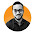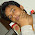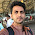# How to find even and odd number in Java - Program tutorial example

Even and Odd number check Java Example
There are many ways to find if a number is even or odd in Java but before moving into technical details on finding even and odd number in Java let's what is even and odd number in terms of Mathematics. Any number which is completely divisible by 2 is called even number while number which is not completely divisible by 2 is called odd number. If you think in terms of remainder than in the case of even number, the remainder is zero while in the case of odd number remainder will be 1. zero is considered as an even number in maths. We can use this property to find whether a number is even or odd in Java. Java has a remainder operator also called modules operation denoted by % which does exactly same. it returns remainder as a result of the division. here is a Java sample program of finding even and odd number using remainder and bitwise AND operator in Java.

## Java program to find even and odd number in JavaAs I said there are multiple ways to check if a number is even or not in Java and if a number is not even then it certainly be odd. Like you can use division operator in a loop and start from 1 keep multiplying it by 2 until you cross the number if number matches than its an even number otherwise it's an odd number.

Another way of finding even and an odd number is by using the bitwise operator in Java. In binary format, the even number has there LSB always zero while the odd number has there LSB as 1 by using this information and bitwise & operator we can find if a number is even or odd in Java.

By the way, in this example, we will see two ways to check if a number is odd or even, first by using remainder operator and second by using bitwise AND operator in Java.

package example;

import java.util.Scanner;

/**
* Java program to find if a number is even or odd in Java or not. This Java program
* example demonstrate two ways to check if number is even or odd or not, first example
* uses modulus or remainder operator denoted by % to see if number is even or not
* and second operator uses Bitwise AND operator to find if the number is even or odd in Java.
*
* @author Javin Paul
*/

public class EvenOddTest{

public static void main(String args[]){

//scanner to get input from user
Scanner console = new Scanner(System.in);

System.out.printf("Enter any number : ");

//return the user input as integer
int number = console.nextInt();

//if remainder is zero than even number
if((number %2)==0){
System.out.printf("number %d is even number %n" , number); //%d -decimal %n new line

} else{
//number is odd in Java
System.out.printf("number %d is odd number %n", number);
}

//Finding Even and Odd number using Bitwise AND operator in Java.

System.out.printf("Finding number if it's even or odd using bitwise AND operator %n");

//For Even numbers
//XXX0
//0001 AND
//0000
if( (number&1) == 0){
System.out.printf("number %d is even number %n" , number);
}else{
System.out.printf("number %d is odd number %n", number);
}

}

}
Output:
Enter any number: 17
number 17 is an odd number
Finding number if its even or odd using bitwise AND operator
number 17 is an odd number
Enter any number: 12
number 12 is an even number
Finding number if its even or odd using bitwise AND operator
number 12 is an even number

That’s all on How to check if a number is even or odd in Java. Surely there are many other ways to check if a number is odd or even and you can certainly find more innovative and creative way of doing it but this is something very useful to know.  Sometimes interviewer twists it a little bit and said how do you check if a number is even or odd without using arithmetic operator and remainder operator, well bit-wise AND is your option which is also fastest method to check even and odd in Java.

Further Learning
Data Structures and Algorithms: Deep Dive Using Java
Java Fundamentals: The Java Language
Complete Java Masterclass

1.now how to solve that using switch

1.//ODD EVEN, USING SWITCH
import java.util.Scanner;

class Parimpar
{
public static void main(String args[])
{
Scanner input = new Scanner(System.in);
int number;
int oddEven;

System.out.println("Ingresa un numero: ");
number = input.nextInt();

oddEven = number%2;

switch (oddEven)
{
case 0:
System.out.println(number+" es par.");

break;

case 1:
System.out.println(number+" es impar.");

break;
}
}
}

2.please explain detail whats the manning of this code: If((number&1)==0)

3.Whats the meaning of :

If((number&1)==0)

And how does it work. Can anyone explain it step by step?

1.lets say our number is even.
when you write its binary form then always you get this pattern X.....XXX0.
i.e the 1st low order bit is always zero and here x may be 0/1.
now take binay of 1-it is 0.....01
take AND operation of both..according to this rule
1&1=1
0&0=0
1&0=0
0&1=0
so
XXX0
0001
-------
0000
now convert this again into its equivalent decimal nmber so it is zero...
conclusion:
if(any number&1==0):
number is even.
else
numbet is odd.

4.Just use it:

boolean isEven(int number) {
return number % 2 == 0;
}

no need switch statement.

1.Try it :

import java.io.DataInputStream;
import java.io.IOException;

public class Even_Odd1 {

static boolean eveOdd(int a){
if(a%2==0)//return true;
System.out.println("The statement is");
else return false;
return true;
}

static String evodd(int a){
if(a%2==0)return "This is an Even number";
else return "This is an Odd number";
}

public static void main(String[] args) throws NumberFormatException, IOException {
DataInputStream d=new DataInputStream(System.in);

boolean b=eveOdd(a);
System.out.println(b);
String s=evodd(a);
System.out.println(s);

}

}

5.You can use a fact that every odd number have 1 at the end of its binary representation so it looks like ???????1 where ? can be either 0 or 1. Here is how you can check it with binary AND -> &

public static boolean isEven(int num) {
return (num & 1) == 0;
}
It works like this:

for odd numbers

????????1 -> any odd number
000000001 -> one
AND ---------
result 000000001 -> one
for even numbers

????????0 -> any even number
000000001 -> one
AND ---------
result 000000000 -> zero